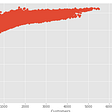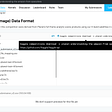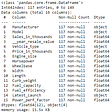# Classification Algorithm Cheat Sheet

In the supervised learning branch of machine learning, there are regression tasks and classification tasks. While regression seeks to answer questions on a continuous scale (ie How much will this house with these features cost?), classification seeks to group our information into predetermined categories. For example, will this person default on their credit card payment, with the groups (or classes) being Yes or No. When confronting a classification problem, there are various algorithms we can use to recognize patterns in our data and correctly classify it. The following is a cheat sheet of these various algorithms, their strengths and weaknesses, as well as some of their important hyperparameters to consider when training your model.

_________________________________________________________________

Algorithm: Logistic Regression

Type: Base

How it Works: Similar to linear regression, this algorithm is represented by an equation (logistic function) that outputs values between 0 and 1. This output represents the probability of the test point belonging to a class, and the class with the higher probability becomes the class prediction.

Assumptions:

• Dependent variable must be binary (in binary logistic regression)
• No multicollinearity between predictors
• Observations are independent of each other
• Independent variables are linearly related to the log of odds
• Usually requires large sample size

Important Hyperparameters:

• C: Inverse of regularization strength. Higher C leads to over overfitting, lower C can lead to underfitting

Pros:

• Relatively quick and efficient
• Provides probability predictions (not just labels)
• C regularization parameter helps to prevent overfitting

Cons:

• Various assumptions to meet
• Works better on large datasets
• Data preparation required (normalizing and scaling)

_________________________________________________________________

Algorithm: K Nearest Neighbors (KNN)

Type: Base

How it Works: Algorithm assumes that the smaller distance between points, the more similar they are. Therefore, a point with certain features and in a certain class, should have the same class as a point with similar features. Point features are stored (as a map of “coordinates”) in the training step, and then during testing, distances between testing and training coordinates are calculated to determine which class the testing point belongs to (based on the classes of the nearest points).

Assumptions:

None

Important Hyperparameters:

• K: Number of neighbors to consider in the determination of class (the higher the K, the higher the risk of underfitting and vice versa)
• Weights: How neighbors should be weighted (for example, should closer neighbors have higher weights than farther neighbors)

Pros:

• Relatively quick and efficient (on smaller datasets)
• Simple and intuitive

Cons:

• Potential for over/underfitting based on value of k
• Exponential runtime (Not good on large datasets and datasets with high dimensionality)
• Data preparation required (scaling)

_________________________________________________________________

Algorithm: Naïve Bayes

Type: Base

How it Works: Algorithm takes Bayes theorem and applies it to multiple variables by assuming that one can estimate the overall probability of being in one class versus another by multiplying the conditional probabilities of the predictors together (given that all the predictors are independent of each other). Model then compares the probabilities of being in each class, and the class with the higher probability becomes the class predicted.

Assumptions:

• All predictors are independent of each other (rarely true, hence the name “naïve” bayes)

Important Hyperparameters:

None

Pros:

• Relatively quick and efficient
• Intuitive
• Provides probability predictions (not just labels)

Cons:

• Dataset must be clean and normalized to get good results
• Not many hyperparameters that can be tuned to improve model

_________________________________________________________________

Algorithm: Decision Tree

Type: Base

How it Works: Trees have nodes that partition the sample space into two or more subspaces (branches). Each node considers a different predictor. The training set is used to construct the tree, moving top down, maximizing information gain (measured with entropy or the Gini index) at the construction of each node. Then each test point is run through the trained tree, where it ends at a leaf node that classifies it.

Assumptions:

None

Important Hyperparameters:

• Criteria: Gini, Entropy — function that measures quality of split
• Max depth: prevents overfitting by limiting the depth of the tree
• Min sample leaf with split: restricts sample leaf size
• Min leaf sample size: restricts size of terminal nodes
• Max leaf nodes: reduces number of leaf nodes
• Max features: maximum number of features to consider when splitting a node

Pros:

• Relatively quick
• Interpretable
• Various hyperparameters to tune that can improve the model

Cons:

• Prone to overfitting if depth and other parameters not specified
• Greedy algorithm — maximizes information gain at each split, but may not be the most effective tree overall

_________________________________________________________________

Algorithm: Random Forrest

Type: Ensemble (tree-based)

How it Works: Algorithm makes a “forest” of different decision trees by bagging (sampling different parts of the data with replacement) and subspace sampling (random subsample of predictors). Each unique tree in the forest then “votes” on the class of the test point, and majority decides the class.

Assumptions:

None

Important Hyperparameters:

• N-estimators: Number of trees in forest
• Criteria: Gini, Entropy — function that measures quality of split
• Max depth: prevents overfitting by limiting the depth of each tree
• Min sample leaf with split: restricts sample leaf size
• Min leaf sample size: restricts size of terminal nodes
• Max leaf nodes: reduces number of leaf nodes
• Max features: maximum number of features to consider when splitting a node

Pros:

• Higher accuracy than regular decision tree due to aggregation of predictions
• More resilient to overfitting through use of diverse set of trees (subspaces of predictors and data) to make predictions
• Interpretable

Cons:

• More computationally expensive than basic models
• High memory usage

_________________________________________________________________

Type: Ensemble (tree-based)

How it Works: Boosting algorithm that uses a tree as a base structure. Works by training a single weak learner (tree) on a subset of data (bag), then identifying the samples the weak learner got wrong. The algorithm increases the weight of samples that the learners misclassify, thereby increasing the likelihood these hard samples will be sampled into the bag to train the next learner. As the iterations continue, there will be more hard samples in the bag to train the weak learners on, thus increasing the likelihood the learner will create a meaningful split to classify the hard example. This is an iterative process that continues until a predetermined stopping condition is met or until the model’s performance plateaus.

Assumptions:

None

Important Hyperparameters:

• Base estimator: default is tree
• N estimators: Number of boosting stages
• Learning rate: Shrinks contribution of each estimator to maximize likelihood of arriving at optimal values

Pros:

• Higher accuracy than base methods due to aggregation of predictions
• Resilient to overfitting (since each individual weak learner is too simple to overfit)

Cons:

• Computationally expensive

_________________________________________________________________

Type: Ensemble (tree-based)

How it Works: Boosting algorithm that uses a tree as a base structure. Works by training a single weak learner (tree), then identifying the samples the weak learner got wrong. The algorithm calculates the residuals for each point to determine the overall loss function for the model, and uses gradient descent to train the next weak learner in a way that works to minimize the loss function (by focusing on the harder samples the model got wrong). This is an iterative process that continues until a predetermined stopping condition is met or until the model’s performance plateaus.

Assumptions:

None

Important Hyperparameters:

• N-estimators: Number of boosting stages
• Learning rate: Shrinks contribution of each tree. Artificially reduces step size in gradient descent, to maximize likelihood of landing on optimal values for cost function
• Max depth: prevents overfitting by limiting the depth of the tree
• Min sample leaf with split: restricts sample leaf size
• Min leaf sample size: restricts size of terminal nodes
• Max leaf nodes: reduces number of leaf nodes
• Max features: maximum number of features to consider when splitting a node

Pros:

• Higher accuracy than base methods due to aggregation of predictions
• Resilient to overfitting (since each individual weak learner is too simple to overfit)

Cons:

• Computationally expensive

_________________________________________________________________

Algorithm: XGBoost

Type: Ensemble (tree based)

How it Works: Implementation of gradient boosted trees, but optimized for speed and performance.

Assumptions:

None

Important Hyperparameters:

• Base estimator: tree or linear functions (for regression tasks)
• Max depth: maximum depth of tree
• Eta (learning rate): step shrinkage to prevent overfitting
• Gamma (minimum split loss): Minimum loss reduction required to further partition leaf (larger gamma means more conservative algorithm).

Pros:

• Known for high accuracy
• Quicker and more efficient than other ensemble algorithms

Cons:

• More computationally complex than other basic algorithms

_________________________________________________________________

Algorithm: Support Vector Machines

Type: Base

How it Works: Algorithm seeks to find the separation line (plane in higher dimensions) that maximizes the distance between classes. For boundaries that cannot be represented linearly, a kernel is used. a kernel creates nonlinear combinations of original features and projects them onto a higher dimensional space, where classes can then be separated.

Assumptions:

None

Important Hyperparameters:

• C: Higher C leads to over overfitting (more precise), lower C can lead to underfitting (bigger margin)
• Kernel: Type of kernel to use for feature transformation, including Linear, RBF, Polynomial, Sigmoid

Pros:

• Higher accuracy than base methods
• Slack component “C” allows one to balance over and underfitting

Cons:

• Computationally expensive
• Data preparation necessary (scaling)

Sources:

• SciKit Learn Documentation
• XGBoost Documentation
• HolyPython.com

--

--

--

## More from Sarah Wright

Love podcasts or audiobooks? Learn on the go with our new app.

## Visualizing Correlation in a Dataset## Optimize problems using Simplex Method and Python## Report of our work for the Data Science Academy, an Ynov Aix Campus startup.## Introducing Flows: from data to AI without a single line of code## Understand Audio Data with Computer Vision Background## Data testing: requirements and levels## 2017 printable calendar## HANDLING MISSING VALUES## Day 20 of #66DaysOfDataChallenge